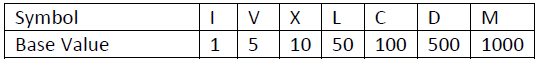# Aromatic-Numbers-芳香数

2012年的题目，网上都是C++和python的代码（老题目了），没看到Java写的…

http://mmhs.ca/ccc/index.htm

https://www.cemc.uwaterloo.ca/contests/computing/2012/index.html

python答案：http://mmhs.ca/ccc/2012/s22012.txt

Try Catch Finally：https://www.cnblogs.com/ECJTUACM-873284962/p/7527052.html

Problem Description

​ This question involves calculating the value of aromatic numbers which are a combination of Arabic digits and Roman numerals. An aromatic number is of the form ARARAR . . . AR, where each A is an Arabic digit, and each R is a Roman numeral.

​ Each pair AR contributes a value described below, and by adding or subtracting these values together we get the value of the entire aromatic number.

​ An Arabic digit A can be 0, 1, 2, 3, 4, 5, 6, 7, 8 or 9.

​ A Roman numeral R is one of the seven letters I, V, X, L, C, D, or M.

​ Each Roman numeral has a base value:​ The value of a pair AR is A times the base value of R. Normally, you add up the values of the pairs to get the overall value. However, wherever there are consecutive symbols ARA’R’ with R’ having a strictly bigger base value than R, the value of pair AR must be subtracted from the total, instead of being added.

​ For example, the number 3M1D2C has the value 3 ∗ 1000 + 1 ∗ 500 + 2 ∗ 100 = 3700 , and 3X2I4X has the value 3 ∗ 10 − 2 ∗ 1 + 4 ∗ 10 = 68.

​ Write a program that computes the values of aromatic numbers.

Input Specification
The input is a valid aromatic number consisting of between 2 and 20 symbols.

Output Specification
The output is the decimal value of the given aromatic number.

• Sample Input 1
3M1D2C
Output for Sample Input 1
3700
• Sample Input 2
2I3I2X9V1X
Output for Sample Input 2
-16

:D 一言句子获取中...